# Periodic Solutions

## Periodic Solutions

Periodic solutions of equations are solutions that describe regularly repeating processes. In such branches of science as the theory of oscillations and celestial mechanics, periodic solutions of systems of differential equations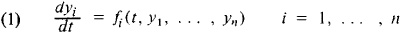are of special interest. A periodic solution yi = Φi (t) of (1) consists of periodic functions of t that have the same period. In other words,

Φi (t + τ) = Φi (t)

for all t and for some τ > 0; τ is called the period of the solution. If the system (1) is autonomous—that is, the functions fi = Fi (y1, …, yn), i = 1, …, n, do not explicitly depend on t —then to the periodic solutions there correspond closed trajectories in the phase space (y1, …, yn). A degenerate form of such trajectories are the equilibrium, or critical, points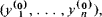where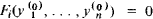The critical points correspond to trivial (constant) periodic solutions. The problem of finding nontrivial periodic solutions has been solved only for special types of differential equations.

Systems of two equations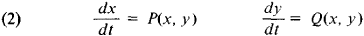are of particular importance in the theory of nonlinear oscillations. Their phase space is the xy-plane. The critical points of system (2) can be found from the system of equations P (x, y) = 0, Q (x, y) = 0. System (2) does not admit of nontrivial periodic solutions if P′x (x, y) + Q′y (x, y) = 0 (Bendixson’s criterion).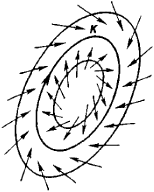Figure 1

The usual method of finding nontrivial periodic solutions of system (2), if they exist, is to construct a bounded annular region K (see Figure 1) such that all trajectories enter it as t → + ∞ or t → – ∞. If k does not contain critical points of system (2), it necessarily contains a closed trajectory that corresponds to a nontrivial periodic solution (the Poincaré-Bendixson theorem). Another approach to finding periodic solutions is provided by the study of the behavior of solutions in neighborhoods of critical points: in the neighborhood of a center, the integral curves of system (2) are closed and correspond to nontrivial periodic solutions.

### REFERENCES

Nemytskii, V. V., and V. V. Stepanov. Kachestvennaia teoriia differentsial’nykh uravnenii, 2nd ed. Moscow-Leningrad, 1949.
Andronov, A. A., A. A. Vitt, and S. E. Khaikin. Teoriia kolebanii, 2nd ed. Moscow, 1959.
Stoker, J. Nelineinye kolebaniia v mekhanicheskikh i elektricheskikh sistemakh, 2nd ed. Moscow, 1953. (Translated from English.)
References in periodicals archive ?
Their topics include friction laws in modeling dynamical systems, the smooth approximation of discontinuous stick-slip solutions, impacts in the chaotic motion of a particle on a non-flat billiard, almost periodic solutions for jumping discontinuous systems, and controlling stochastically excited systems with an approximate discontinuity.
It approved the preparation of a comprehensive study to plan the transportation of goods in the Sultanate, to provide periodic solutions for the existing problems of truck transport, as well as long-term solutions.
Almost periodic, asymptotically almost periodic, and pseudo-almost periodic solutions for differential, and difference equations arise naturally in biology, economics and physics [9, 10, 11, 12, 13].
Therefore, the stability problem for SICNNs has been one of the most active areas of research and there exist some results on the existence and stability of periodic and almost periodic solutions for the SICNNs with delays [2-11].
Modulation equations were derived for the amplitude and phase angle of the periodic solutions and serve as normal forms of the governing equations representing the nonlinear dynamics of the ballend milling system considered.
CHEBAN, Asymptotic Almost Periodic Solutions of Differential Equations, Hindawi Publishing Corporation, New York 2009.
Determination of periodic solutions for the motion of a particle on a rotating parabola by means of optimal homotopy asymptotic method J.
Abdou: New periodic solutions for nonlinear evolution equations using Exp-function method, Chaos Soliton Fract.
the existence of periodic solutions of approximate systems, some a priori estimates for solutions of approximate equations and convergence of solutions.
In the present paper, we obtain an approximate expression for the periodic solutions to two practical cases [25, 26] of nonlinear SODF oscillation systems, namely oscillation of current-carrying wire in a magnetic field and the model of bucking of a column by means of iteration perturbation method (IPM), variational approach (VA), and perturbation expansion method (PEM).
This approach allowed tracking the periodic solutions of the system defining the stability of the fixed points at every step.
Divided into two sections covering application of information and computing, and applied mathematics and modeling, the work features papers addressing such topics as computational intelligence performance in cloud computing applications, GIS based on network analysis models, computable analysis of boundary-value problems, and global periodic solutions to predator-prey diffusive system problems.

Site: Follow: Share:
Open / Close